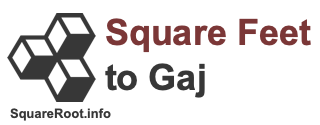99 square feet in gajHere we will explain and show you how to convert 99 square feet to gaj. Before we continue, note that 99 square feet to gaj is the same as 99 sq ft to gaj, 99 ft2 to gaj, and 99 ft² to gaj.

Square feet and gaj are both measurements of an area, like a field or land area. One square foot is equal to 0.112188212713609 gaj. Therefore, we can make the following square feet to gaj formula:

square feet × 0.112188212713609
= gaj

Below is the math using our square feet to gaj formula to get 99 square feet in gaj.

= square feet × 0.112188212713609
= 99 × 0.112188212713609
= 11.1066330586473
99 square feet ≈ 11.11 gaj

We have seen real estate brokers and others rounding a square foot to 1/9 gaj. Consequently, they simply multiply 99 square feet by 1/9 to get it converted to gaj. In that case, this is how you would convert 99 square feet to gaj:

square feet × 1/9 = gaj
99 × 1/9 = 11
99 square feet ≈ 11.00 gaj

Square Feet to Gaj Converter
Please enter another square feet area in the box below to have it converted to gaj.

Convert  square feet to gaj.

100 square feet in gaj
Here is the next area in square feet on our list that we have converted to gaj for you.